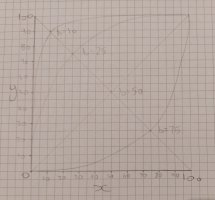# chart plotting

#### daarsk

##### New member
I wasn't sure exactly where to post this, so I put here..
I am also far from a math scholar, so my terminology and knowledge may not be great.

I would like to plot a smooth line from 0,0 to 100,100 that intercepts (b), where b is on a line from (x=100-y). see belowEssentially, I am looking for (x), where (y) and (b) is given.
(y) is 0 - 100
(b) is (x) where (x = 100-y)
for example. where (y = 90) and (b = 10), (x) will equal 10.
but if (y = 70) and (b = 10), what is x?

In the end, I would love an equation that is something like (x = y something something b something something), for example.

I hope I have explained this well. And highly appreciate any help.
fyi, if the picture attached needs to be better quality, I can redraw. just ask. Thanks.

#### Dr.Peterson

##### Elite Member
I would like to plot a smooth line from 0,0 to 100,100 that intercepts (b), where b is on a line from (x=100-y). see below

View attachment 29123

Essentially, I am looking for (x), where (y) and (b) is given.
(y) is 0 - 100
(b) is (x) where (x = 100-y)
for example. where (y = 90) and (b = 10), (x) will equal 10.
but if (y = 70) and (b = 10), what is x?

In the end, I would love an equation that is something like (x = y something something b something something), for example.

On the graph, it appears that x = b. That is true in the example you give.

Also, this does not seem to involve "plotting a smooth line". So I don't think you've said what you want to do.

If you are given a point (b, 100-b) on that diagonal line, and want to find a smooth function y = f(x) that passes through that point as well as (0,0) and (100,100), then you still need to decide what kind of function you want. Your curves appear to be symmetrical about the diagonal line; one class of such functions would be shifted reciprocal functions. But the answer depends partly on what you will do with the curve you get! If you are modeling some real-life behavior, for example, we need to know what it is.

•Jomo and lev888